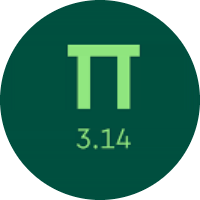# Learning the Basic Geometric Measurement

In this quiz, you will get to know many concepts about Geometric measurement. You will learn about the perimeter and area of the different triangles, squares, rectangles, trapezoids, compound figures, and cubes.

Start Quiz

Look at this rectangle. Find the value of m.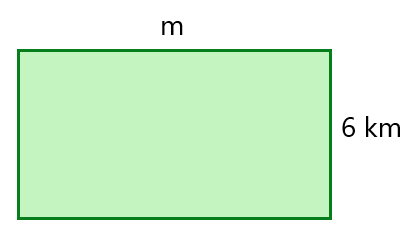The perimeter of the rectangle = 34 km

m = 12

m = 11

m = 10

Find the area of the rectangle.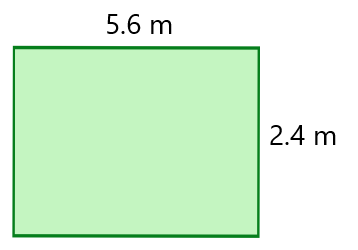12.24 sq. m

13.44 sq. m

15.24 sq. m

What is the area of the parallelogram?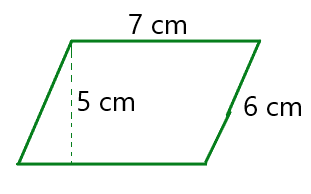42

30

35

Find the area of the trapezoid?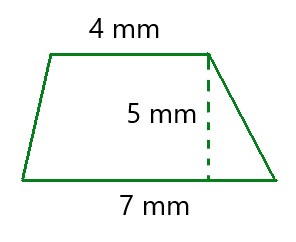27.5 sq. mm

35 sq. mm

32.5 sq. mm

Find the length of the rectangle.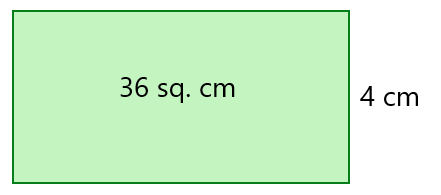6 cm

9 cm

8 cm

Find the area of the shape.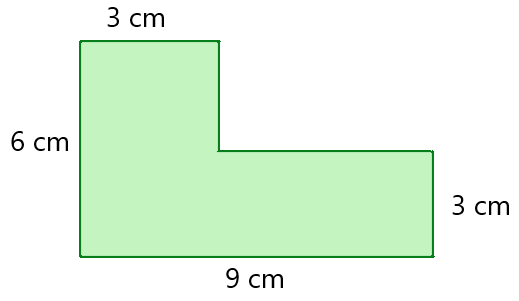54 sq. cm

36 sq. cm

45 sq. cm

What is the area of the shaded region?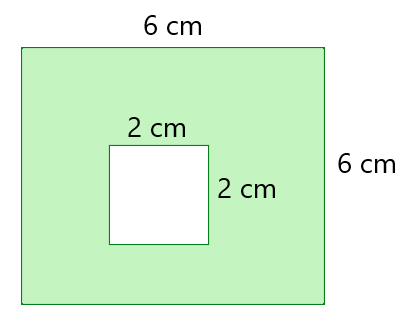4 sq. cm

36 sq. cm

32 sq. cm

What is the area of the shaded region?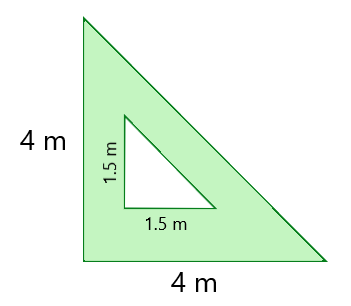6.875 sq. m

5.675 sq. m

4.875 sq. m

Rectangles I and II have the same perimeters. What is the area of the II rectangle?36 sq. cm

18 sq. cm

22 sq. cm

What is the volume of the cube?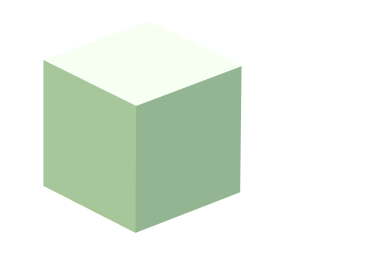each side is equal to 1.6 mm.

4.096

2.048

2.56

Quiz/Test Summary
Title: Learning the Basic Geometric Measurement
Questions: 10
Contributed by: